Chemical Equations Reactions Chemical Equations 4 Als 3

• Slides: 44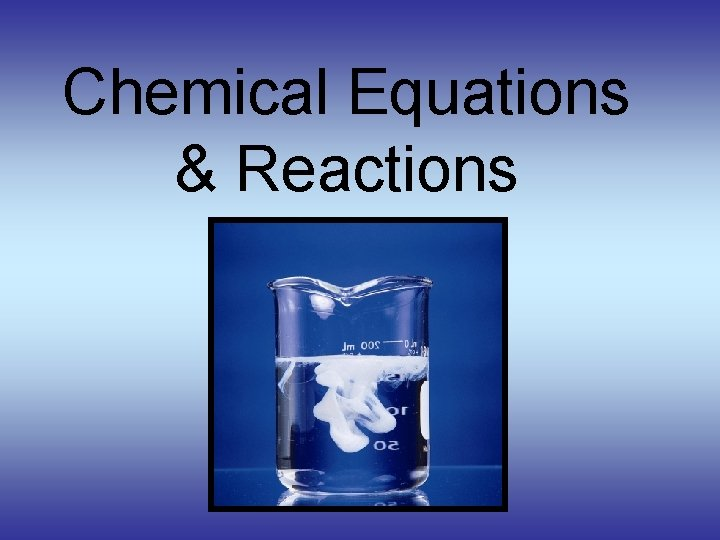Chemical Equations & Reactions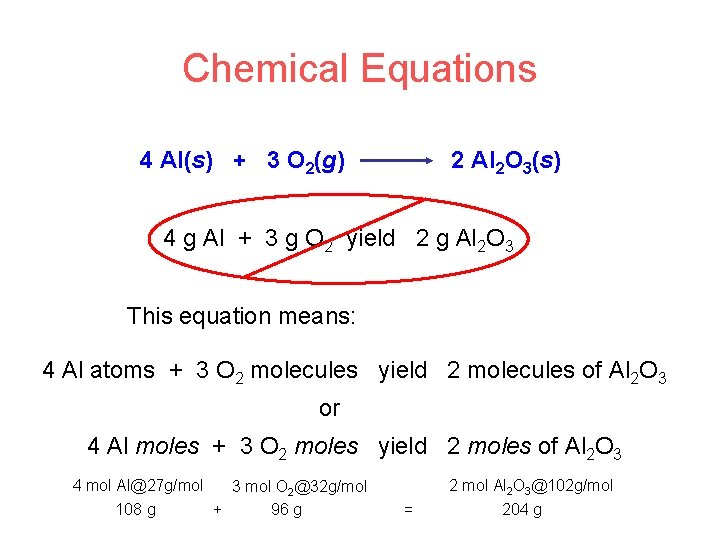Chemical Equations 4 Al(s) + 3 O 2(g) 2 Al 2 O 3(s) 4 g Al + 3 g O 2 yield 2 g Al 2 O 3 This equation means: 4 Al atoms + 3 O 2 molecules yield 2 molecules of Al 2 O 3 or 4 Al moles + 3 O 2 moles yield 2 moles of Al 2 O 3 4 mol [email protected] g/mol 3 mol O [email protected] g/mol 108 g + 96 g 2 mol Al 2 O [email protected] g/mol = 204 gVisualizing a Chemical Reaction 2 Na 10 mole Na ___ + Cl 2 5 mole Cl 2 ___ 2 Na. Cl 10 ? mole Na. Cl ___Types of Chemical Reactions Synthesis (combination) reaction A + B AB AB A + B Decomposition reaction ASingle-replacement reaction BDouble-replacement reaction Combustion reaction (of a hydrocarbon) Ause A + BC AC + B element compound element AB + CD AD + CB All compounds… Cx. Hy + O 2 CO 2 + H 2 O activity series to predict products/reactivity Buse solubility chart to predict products/reactivity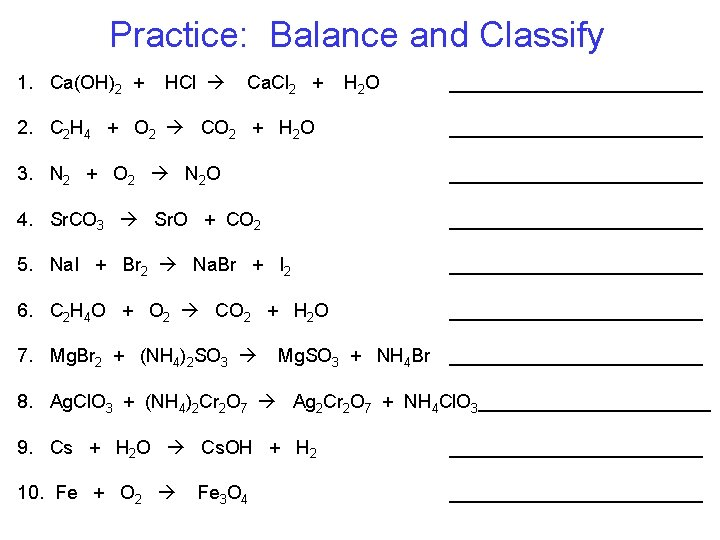Practice: Balance and Classify 1. Ca(OH)2 + HCl Ca. Cl 2 + H 2 O ____________ 2. C 2 H 4 + O 2 CO 2 + H 2 O ____________ 3. N 2 + O 2 N 2 O ____________ 4. Sr. CO 3 Sr. O + CO 2 ____________ 5. Na. I + Br 2 Na. Br + I 2 ____________ 6. C 2 H 4 O + O 2 CO 2 + H 2 O ____________ 7. Mg. Br 2 + (NH 4)2 SO 3 ____________ Mg. SO 3 + NH 4 Br 8. Ag. Cl. O 3 + (NH 4)2 Cr 2 O 7 Ag 2 Cr 2 O 7 + NH 4 Cl. O 3___________ 9. Cs + H 2 O Cs. OH + H 2 ____________ 10. Fe + O 2 ____________ Fe 3 O 4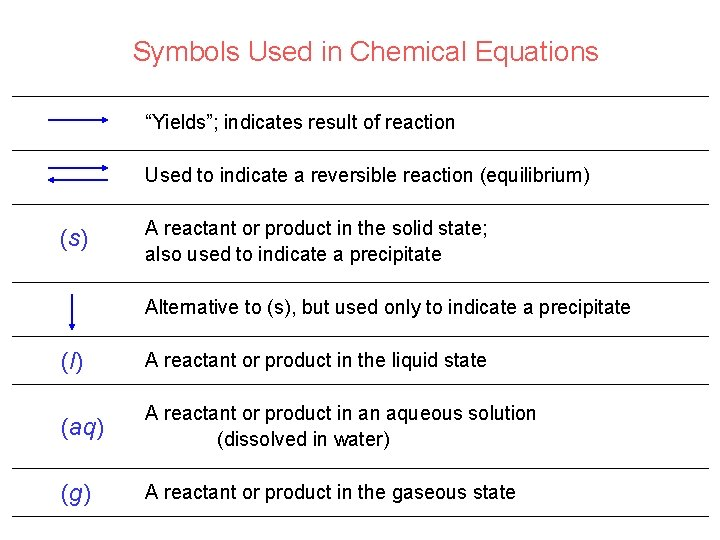Symbols Used in Chemical Equations “Yields”; indicates result of reaction Used to indicate a reversible reaction (equilibrium) (s) A reactant or product in the solid state; also used to indicate a precipitate Alternative to (s), but used only to indicate a precipitate (l) A reactant or product in the liquid state (aq) A reactant or product in an aqueous solution (dissolved in water) (g) A reactant or product in the gaseous state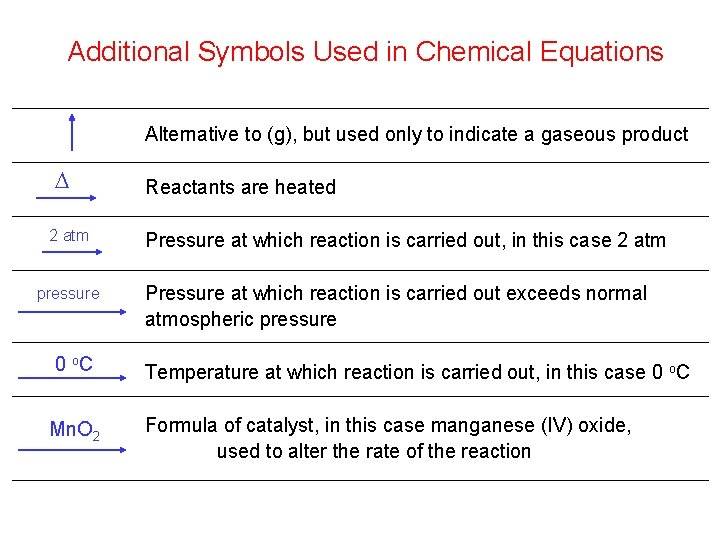Additional Symbols Used in Chemical Equations Alternative to (g), but used only to indicate a gaseous product D 2 atm pressure Reactants are heated Pressure at which reaction is carried out, in this case 2 atm Pressure at which reaction is carried out exceeds normal atmospheric pressure 0 o. C Temperature at which reaction is carried out, in this case 0 o. C Mn. O 2 Formula of catalyst, in this case manganese (IV) oxide, used to alter the rate of the reaction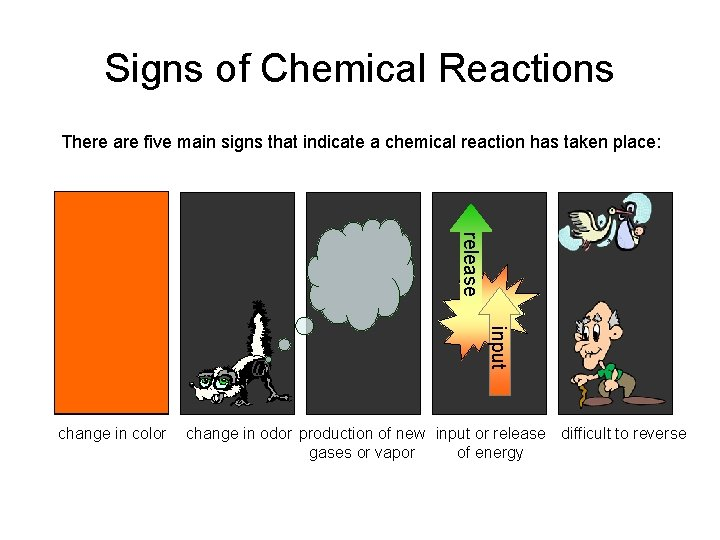Signs of Chemical Reactions There are five main signs that indicate a chemical reaction has taken place: release input change in color change in odor production of new input or release difficult to reverse gases or vapor of energy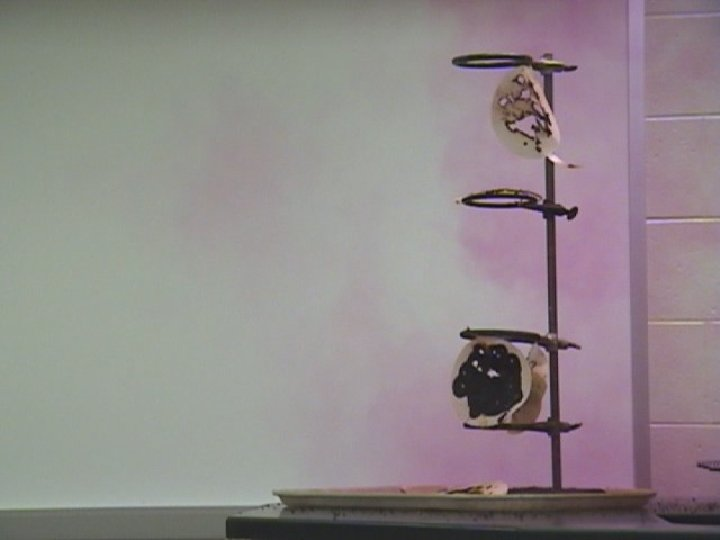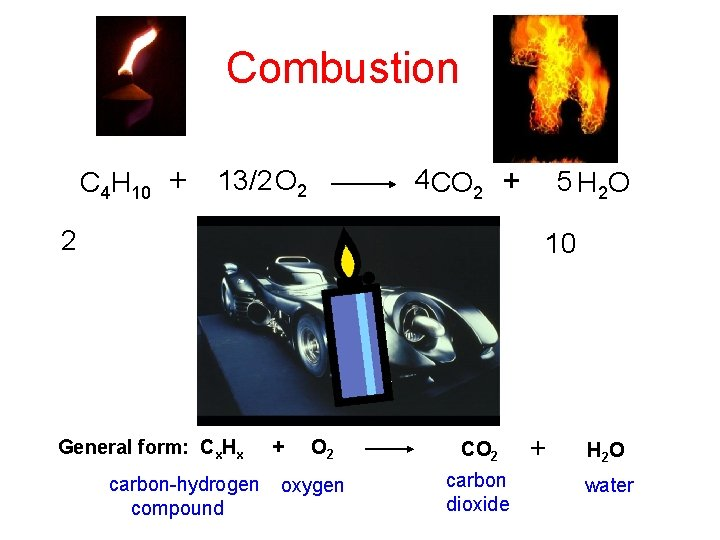Combustion C 4 H 10 + 2 13/2 O 2 4 CO 2 + 13 8 General form: Cx. Hx carbon-hydrogen compound + O 2 oxygen 5 H 2 O 10 CO 2 carbon dioxide + H 2 O waterWriting Equations Practice Write a balanced equation for the reaction between chlorine and solid sodium bromide to produce bromine and solid sodium chloride. 1. Write a word equation for the reaction chlorine + sodium bromide bromine + sodium chloride 2. Write the correct formulas for all reactants and products, (with correct phases of matter) 3. Balance the resulting equation Cl 2(g) + 2 Na. Br(s) Br 2(l) + 2 Na. Cl(s)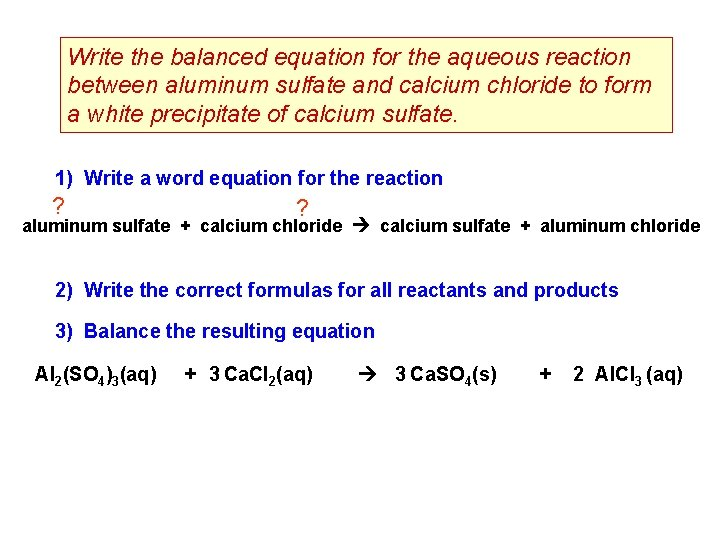Write the balanced equation for the aqueous reaction between aluminum sulfate and calcium chloride to form a white precipitate of calcium sulfate. 1) Write a word equation for the reaction ? ? aluminum sulfate + calcium chloride calcium sulfate + aluminum chloride 2) Write the correct formulas for all reactants and products 3) Balance the resulting equation Al 2(SO 4)3(aq) + 3 Ca. Cl 2(aq) 3 Ca. SO 4(s) + 2 Al. Cl 3 (aq)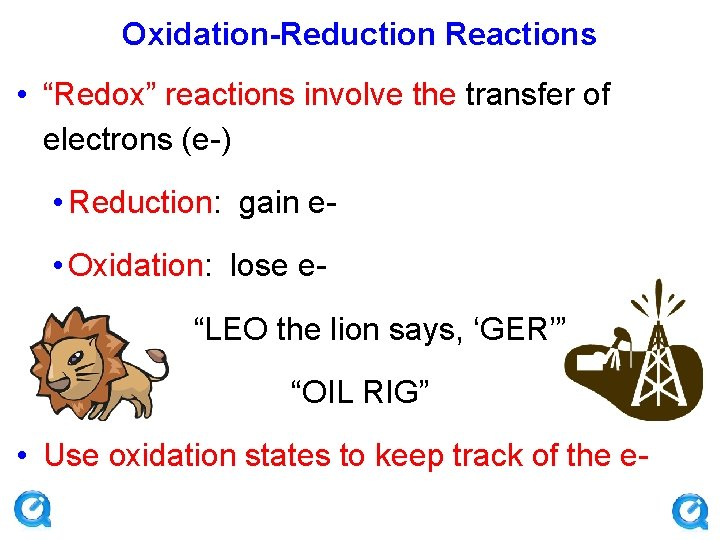Oxidation-Reduction Reactions • “Redox” reactions involve the transfer of electrons (e-) • Reduction: gain e • Oxidation: lose e“LEO the lion says, ‘GER’” “OIL RIG” • Use oxidation states to keep track of the e-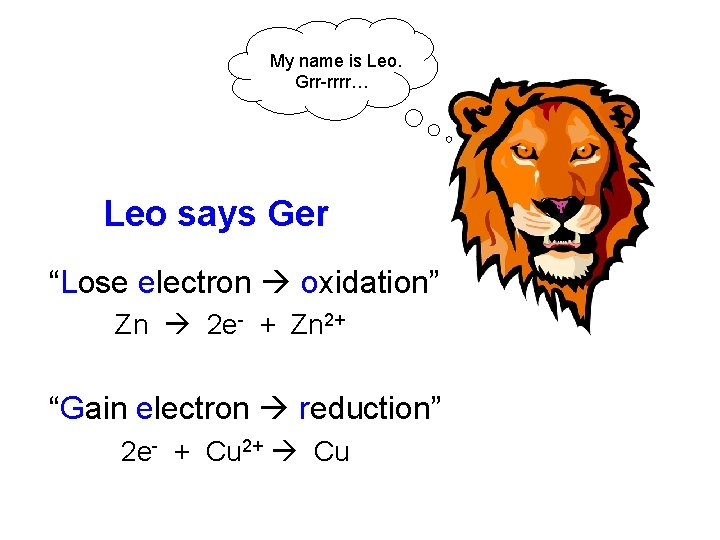My name is Leo. Grr-rrrr… Leo says Ger “Lose electron oxidation” Zn 2 e- + Zn 2+ “Gain electron reduction” 2 e- + Cu 2+ CuAssigning Oxidation States Specific rules for assigning Ox #’s • • • Usually the same charge assigned by the PT H is almost always +1 O is almost always -2 F is always -1 in compounds For elements (H 2, O 2, F 2, Ca, K, etc ) the oxidation state always = 0 Some exceptions do exist!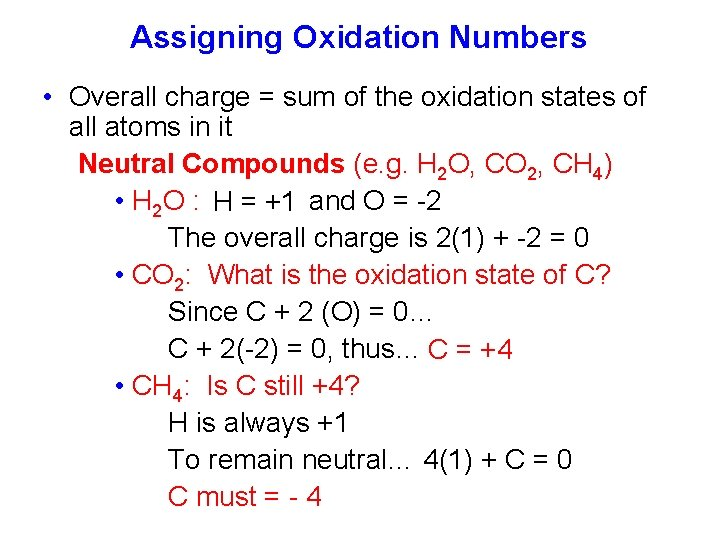Assigning Oxidation Numbers • Overall charge = sum of the oxidation states of all atoms in it Neutral Compounds (e. g. H 2 O, CO 2, CH 4) • H 2 O : H = +1 and O = -2 The overall charge is 2(1) + -2 = 0 • CO 2: What is the oxidation state of C? Since C + 2 (O) = 0… C + 2(-2) = 0, thus… C = +4 • CH 4: Is C still +4? H is always +1 To remain neutral… 4(1) + C = 0 C must = - 4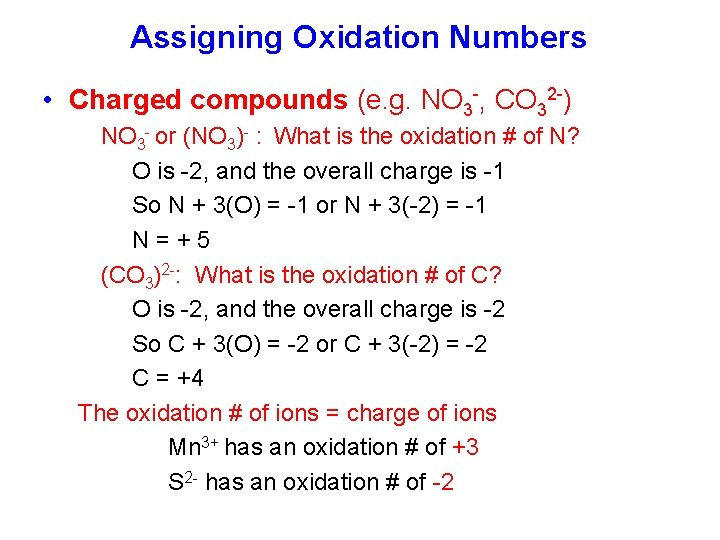Assigning Oxidation Numbers • Charged compounds (e. g. NO 3 -, CO 32 -) NO 3 - or (NO 3)- : What is the oxidation # of N? O is -2, and the overall charge is -1 So N + 3(O) = -1 or N + 3(-2) = -1 N=+5 (CO 3)2 -: What is the oxidation # of C? O is -2, and the overall charge is -2 So C + 3(O) = -2 or C + 3(-2) = -2 C = +4 The oxidation # of ions = charge of ions Mn 3+ has an oxidation # of +3 S 2 - has an oxidation # of -2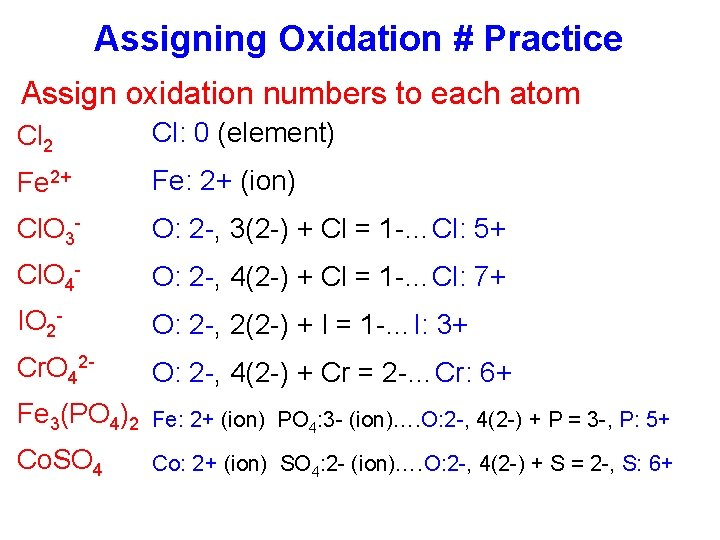Assigning Oxidation # Practice Assign oxidation numbers to each atom Cl 2 Cl: 0 (element) Fe 2+ Fe: 2+ (ion) Cl. O 3 - O: 2 -, 3(2 -) + Cl = 1 -…Cl: 5+ Cl. O 4 - O: 2 -, 4(2 -) + Cl = 1 -…Cl: 7+ IO 2 - O: 2 -, 2(2 -) + I = 1 -…I: 3+ Cr. O 42 - O: 2 -, 4(2 -) + Cr = 2 -…Cr: 6+ Fe 3(PO 4)2 Fe: 2+ (ion) PO 4: 3 - (ion)…. O: 2 -, 4(2 -) + P = 3 -, P: 5+ Co. SO 4 Co: 2+ (ion) SO 4: 2 - (ion)…. O: 2 -, 4(2 -) + S = 2 -, S: 6+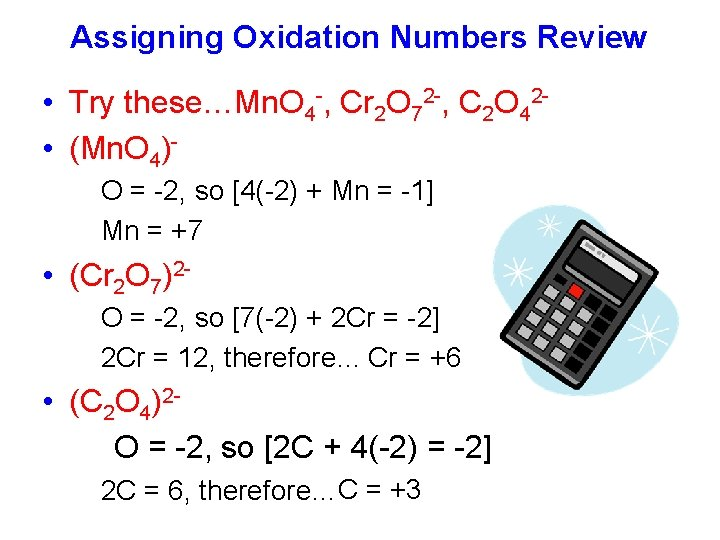Assigning Oxidation Numbers Review • Try these…Mn. O 4 -, Cr 2 O 72 -, C 2 O 42 • (Mn. O 4)O = -2, so [4(-2) + Mn = -1] Mn = +7 • (Cr 2 O 7)2 O = -2, so [7(-2) + 2 Cr = -2] 2 Cr = 12, therefore… Cr = +6 • (C 2 O 4)2 O = -2, so [2 C + 4(-2) = -2] 2 C = 6, therefore…C = +3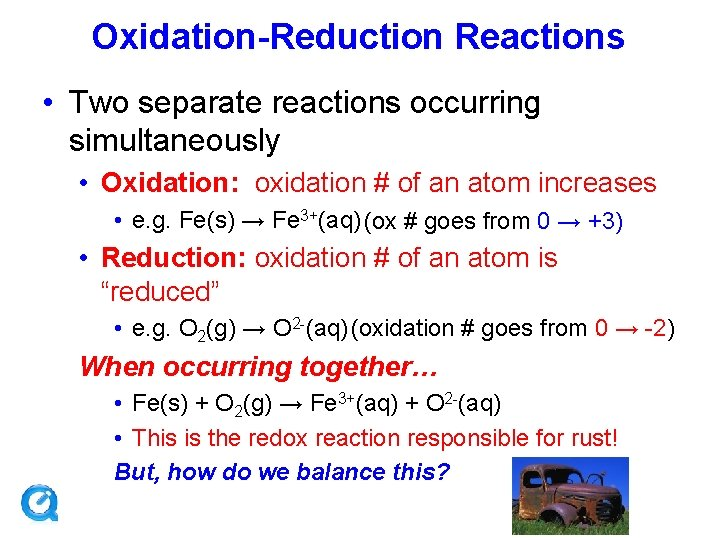Oxidation-Reduction Reactions • Two separate reactions occurring simultaneously • Oxidation: oxidation # of an atom increases • e. g. Fe(s) → Fe 3+(aq) (ox # goes from 0 → +3) • Reduction: oxidation # of an atom is “reduced” • e. g. O 2(g) → O 2 -(aq) (oxidation # goes from 0 → -2) When occurring together… • Fe(s) + O 2(g) → Fe 3+(aq) + O 2 -(aq) • This is the redox reaction responsible for rust! But, how do we balance this?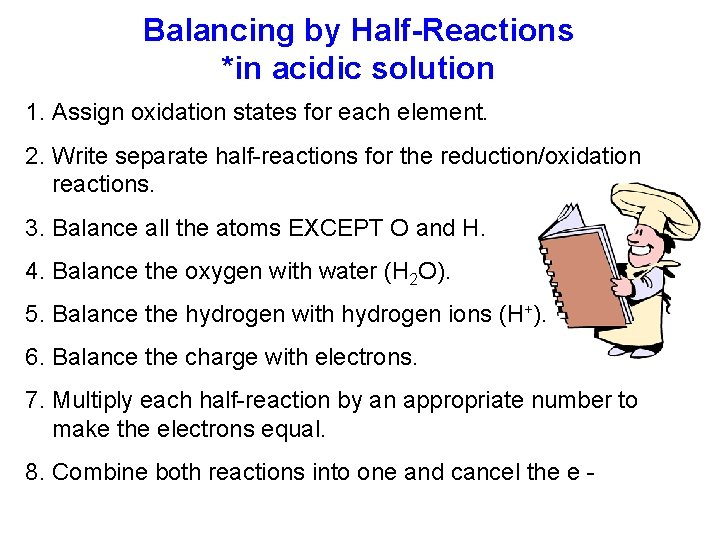Balancing by Half-Reactions *in acidic solution 1. Assign oxidation states for each element. 2. Write separate half-reactions for the reduction/oxidation reactions. 3. Balance all the atoms EXCEPT O and H. 4. Balance the oxygen with water (H 2 O). 5. Balance the hydrogen with hydrogen ions (H+). 6. Balance the charge with electrons. 7. Multiply each half-reaction by an appropriate number to make the electrons equal. 8. Combine both reactions into one and cancel the e -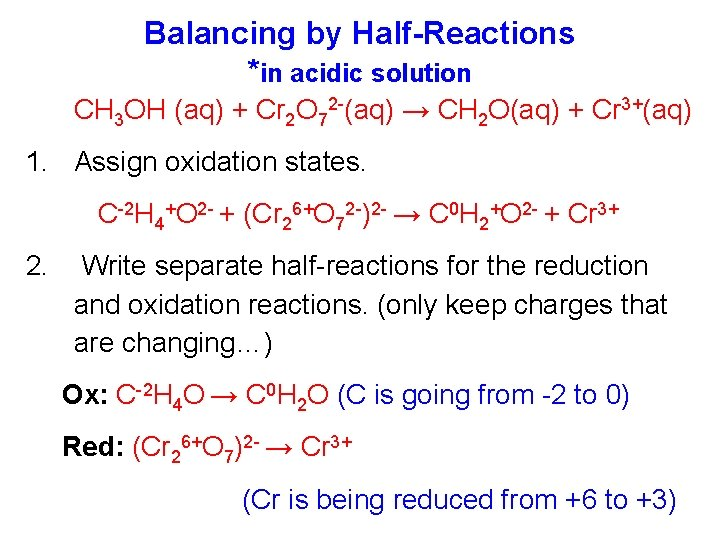Balancing by Half-Reactions *in acidic solution CH 3 OH (aq) + Cr 2 O 72 -(aq) → CH 2 O(aq) + Cr 3+(aq) 1. Assign oxidation states. C-2 H 4+O 2 - + (Cr 26+O 72 -)2 - → C 0 H 2+O 2 - + Cr 3+ 2. Write separate half-reactions for the reduction and oxidation reactions. (only keep charges that are changing…) Ox: C-2 H 4 O → C 0 H 2 O (C is going from -2 to 0) Red: (Cr 26+O 7)2 - → Cr 3+ (Cr is being reduced from +6 to +3)Balancing the half reactions… 3 ( C 2 -H 4 O → C 0 H 2 O + 2 H+ + 2 e- ) Ox: Red: 6 e- +14 H+ +(Cr 26+O 7)2 - → 2 Cr 3+ + 7 H 2 O 3 CH 4 O → 3 CH 2 O + 6 H+ + 6 e 3 CH 4 O + 8 H++ Cr 2 O 72 - → 3 CH 2 O + 2 Cr 3+ + 7 H 2 O 3. 4. 5. 6. 7. 8. For each half reaction, balance all atoms EXCEPT O and H. Balance the oxygen by adding water (H 2 O). Balance the hydrogen by adding hydrogen ions (H+) Balance the charge by adding electrons. …use the oxidation state as a guide Multiply each half-reaction by an appropriate number to make the electrons equal. Add the reactions together and cancel e-/simplify.Practice Balancing Redox Reactions Unbalanced reaction (in acid): Mn. O 4 + Fe 2+ Mn 2+ + Fe 3+ Balanced Reduction half-reaction: 8 H+ + Mn. O 4 + 5 e Mn 2+ + 4 H 2 O Balanced Oxidation half-reaction: 5(Fe 2+ Fe 3+ + e ) Balanced overall reaction: 8 H+ + Mn. O 4 + 5 Fe 2+ Mn 2+ + 5 Fe 3+ + 4 H 2 OBalancing by Half-Reactions *in basic solution 1. Assign oxidation states. 2. Write separate half-reactions for the reduction/oxidation reactions. 3. Balance all the atoms EXCEPT O and H. 4. Balance the oxygen by adding water (H 2 O). 5. Balance the hydrogen by adding H+. 6. Balance the charge by adding electrons. 7. Multiply each half-reaction by an appropriate number to make the electrons equal. 8. Combine both reactions into one and cancel. 9. Add OH- to both sides to cancel out H+ and create H 2 O. Simplify further, if necessary.Balancing by Half-Reactions (in basic solution) Let’s balance a previous example in basic solution Remember, it is all the same steps up to this point 3 CH 4 O + 8 H+ + Cr 2 O 72 - → 3 CH 2 O + 2 Cr 3+ + 7 H 2 O + 8 OH 3 CH 4 O + 8 H 2 O + Cr 2 O 72 - → 3 CH 2 O + 2 Cr 3+ + 7 H 2 O + 8 OH- 3 CH 4 O + H 2 O + Cr 2 O 72 - → 3 CH 2 O + 2 Cr 3+ + 8 OH-Practice Balancing Basic Redox Rxns Unbalanced reaction: Cl. O + Zn Cl- + Zn 2+ Balanced Reduction half-reaction: 2 e- + 2 H+ + Cl. O- Cl- + H 2 O Balanced Oxidation half-reaction: Zn 2+ + 2 e. Balanced overall reaction (acidic): 2 H+ + Cl. O + Zn 2+ + Cl- + H 2 O Balanced overall reaction (basic): H 2 O + Cl. O + Zn 2+ + Cl- + 2 OH-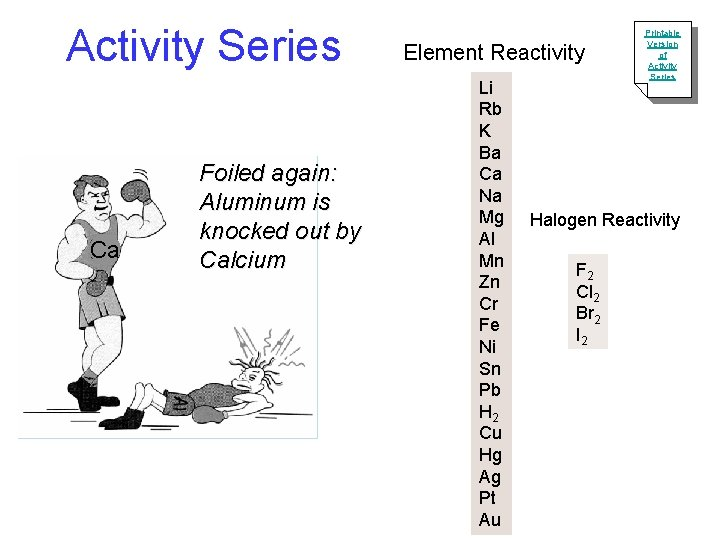Activity Series Ca Foiled again: Aluminum is knocked out by Calcium Element Reactivity Li Rb K Ba Ca Na Mg Al Mn Zn Cr Fe Ni Sn Pb H 2 Cu Hg Ag Pt Au Printable Version of Activity Series Halogen Reactivity F 2 Cl 2 Br 2 I 2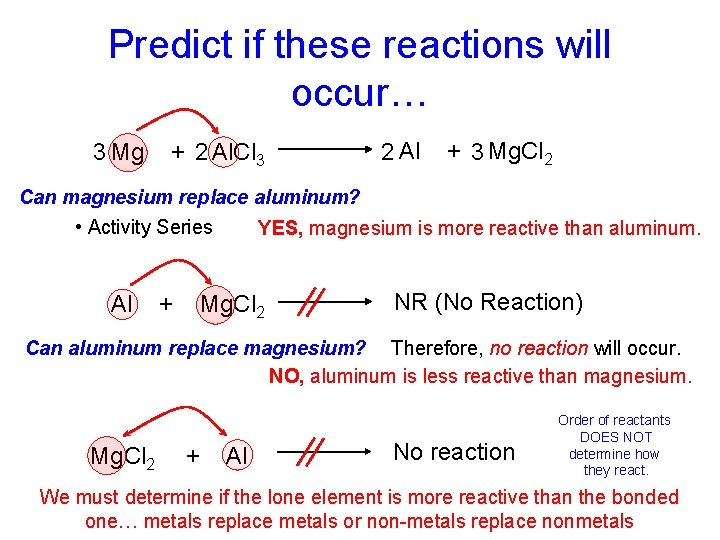Predict if these reactions will occur… 3 Mg + 2 Al. Cl 3 2 Al + 3 Mg. Cl 2 Can magnesium replace aluminum? • Activity Series Al + YES, magnesium is more reactive than aluminum. Mg. Cl 2 NR (No Reaction) Can aluminum replace magnesium? Therefore, no reaction will occur. NO, aluminum is less reactive than magnesium. Mg. Cl 2 + Al No reaction Order of reactants DOES NOT determine how they react. We must determine if the lone element is more reactive than the bonded one… metals replace metals or non-metals replace nonmetals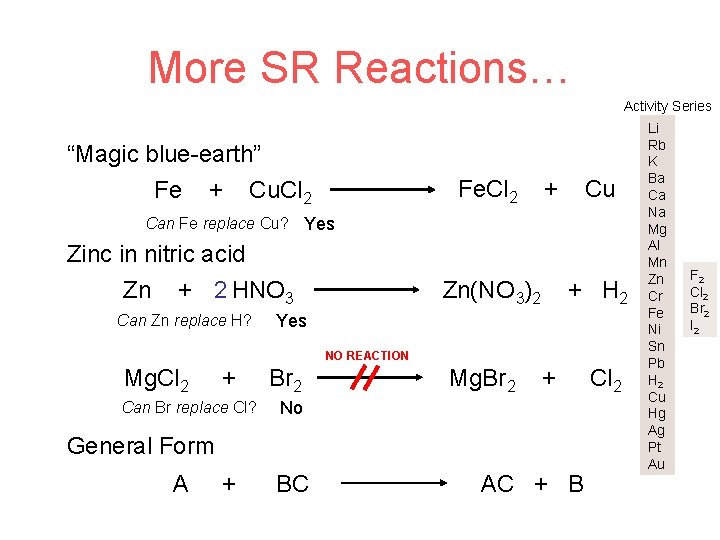More SR Reactions… Activity Series “Magic blue-earth” Fe + Cu. Cl 2 Fe. Cl 2 + Cu Can Fe replace Cu? Yes Zinc in nitric acid Zn + 2 HNO 3 Can Zn replace H? Zn(NO 3)2 + H 2 Yes NO REACTION Mg. Cl 2 + Can Br replace Cl? Br 2 Mg. Br 2 + No General Form A + BC AC + B Cl 2 Li Rb K Ba Ca Na Mg Al Mn Zn Cr Fe Ni Sn Pb H 2 Cu Hg Ag Pt Au F 2 Cl 2 Br 2 I 2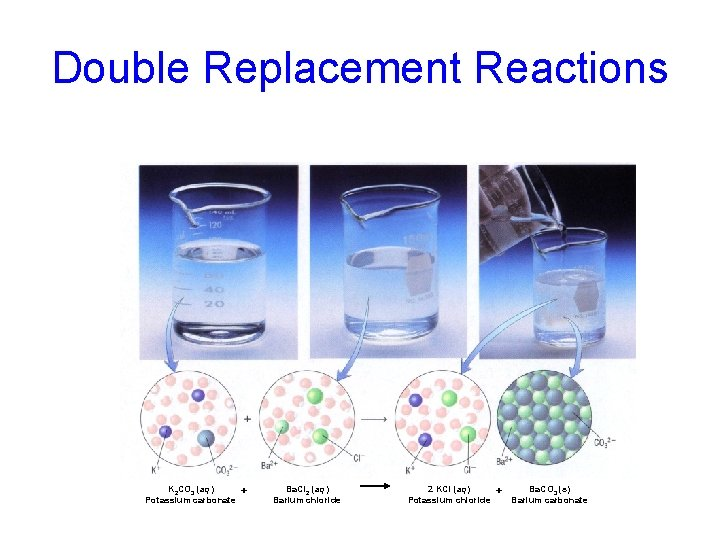Double Replacement Reactions K 2 CO 3 (aq) Potassium carbonate + Ba. Cl 2 (aq) Barium chloride 2 KCl (aq) Potassium chloride + Ba. CO 3 (s) Barium carbonate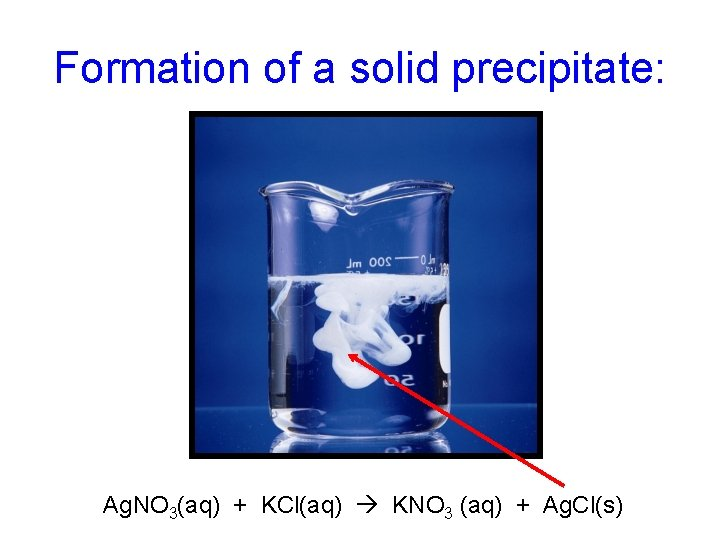Formation of a solid precipitate: Ag. NO 3(aq) + KCl(aq) KNO 3 (aq) + Ag. Cl(s)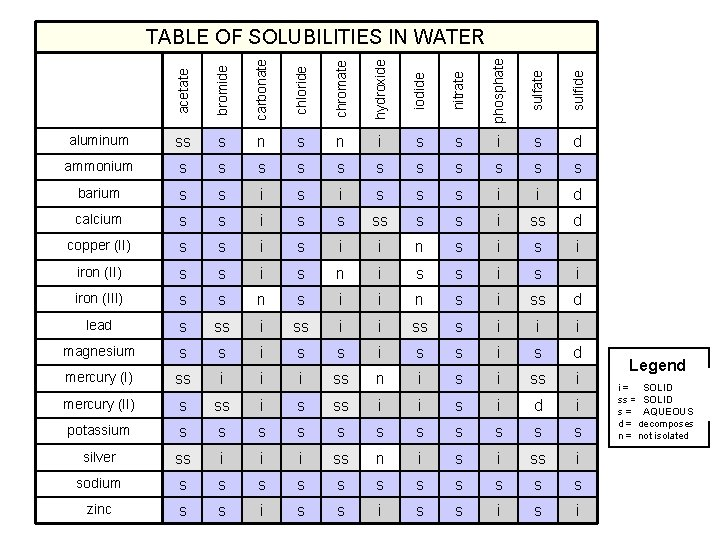acetate bromide carbonate chloride chromate hydroxide iodide nitrate phosphate sulfide TABLE OF SOLUBILITIES IN WATER aluminum ss s n i s s i s d ammonium s s s barium s s i s s s i i d calcium s s i ss d copper (II) s s i i n s i iron (II) s s i s n i s s i iron (III) s s n s i i n s i ss d lead s ss i i ss s i i i magnesium s s i s d mercury (I) ss i i i ss n i ss i mercury (II) s ss i i s i d i potassium s s silver ss i i i ss n i ss i sodium s s s zinc s s i Legend SOLID i = insoluble SOLIDsoluble ss = slightly AQUEOUS s = soluble d = decomposes n = not isolated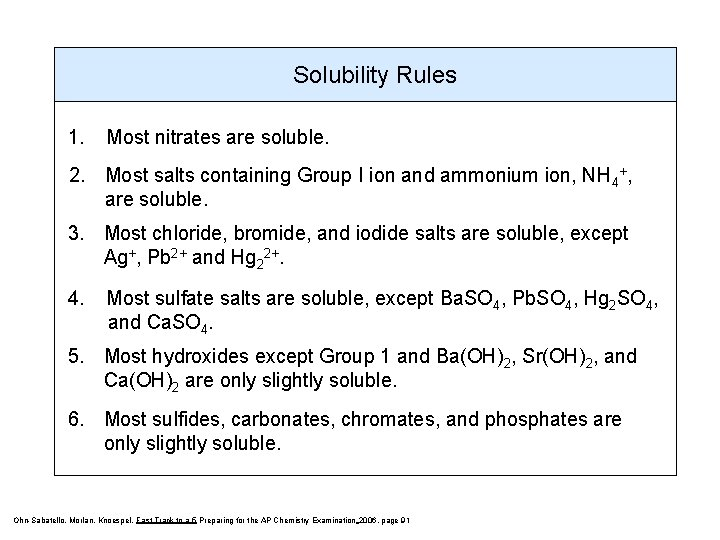Solubility Rules 1. Most nitrates are soluble. 2. Most salts containing Group I ion and ammonium ion, NH 4+, are soluble. 3. Most chloride, bromide, and iodide salts are soluble, except Ag+, Pb 2+ and Hg 22+. 4. Most sulfate salts are soluble, except Ba. SO 4, Pb. SO 4, Hg 2 SO 4, and Ca. SO 4. 5. Most hydroxides except Group 1 and Ba(OH)2, Sr(OH)2, and Ca(OH)2 are only slightly soluble. 6. Most sulfides, carbonates, chromates, and phosphates are only slightly soluble. Ohn-Sabatello, Morlan, Knoespel, Fast Track to a 5 Preparing for the AP Chemistry Examination 2006, page 91Predict if a reaction will occur when you combine aqueous solutions of iron (II) chloride and sodium carbonate… If the reaction does occur, write a Balanced balanced chemical equation showing it (be sure to include phase notation). iron (II) chloride + sodium carbonate Fe 2+ Cl - Na+ CO 32 - Fe. Cl 2 Na 2 CO 3 sodium chloride + iron (II) carbonate Na+ CO 32 - Fe 2+ Cl- Fe. CO 3 (s) Na. Cl (aq) Using the SOLUBILITY TABLE: sodium chloride is soluble iron (II) carbonate is insoluble Fe. Cl 2 (aq) + Na 2 CO 3 (aq) 2 Na. Cl (aq) + Fe. CO 3 (s)Predict if a reaction will occur when you combine aqueous solutions of potassium chloride and sodium nitrate… If the reaction does occur, write a balanced chemical equation showing it (be sure to include phase notation). R N potassium chloride + sodium nitrate K+ Cl KCl(aq) Na+ NO 3 - Na. NO 3 (aq) sodium chloride + potassium nitrate K+ Cl- Na. Cl(aq) Na+ NO 3 - KNO 3(aq) Using the SOLUBILITY TABLE: sodium chloride is soluble potassium nitrate is soluble Notice that nothing has really changed because the ions are still dissolved in water!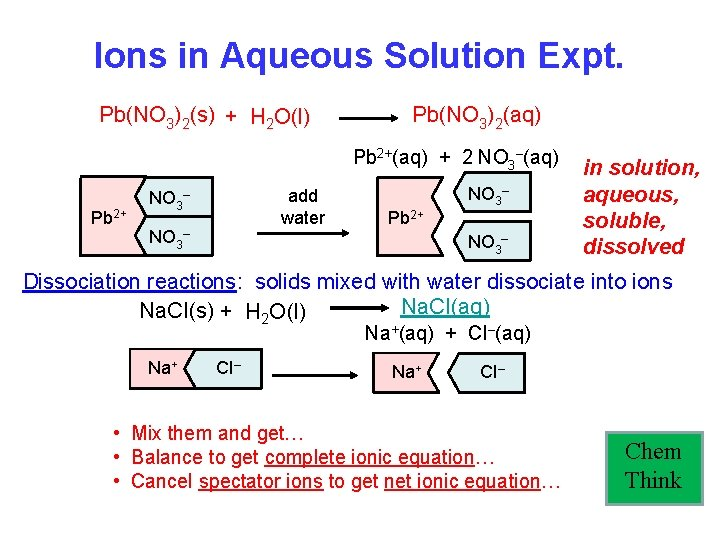Ions in Aqueous Solution Expt. Pb(NO 3)2(s) + H 2 O(l) Pb(NO 3)2(aq) Pb 2+(aq) + 2 NO 3–(aq) Pb 2+ add water NO 3– Pb 2+ NO 3– in solution, aqueous, soluble, dissolved Dissociation reactions: solids mixed with water dissociate into ions Na. CI(aq) Na. CI(s) + H 2 O(l) Na+(aq) + CI–(aq) Na+ CI– • Mix them and get… • Balance to get complete ionic equation… • Cancel spectator ions to get net ionic equation… Chem Think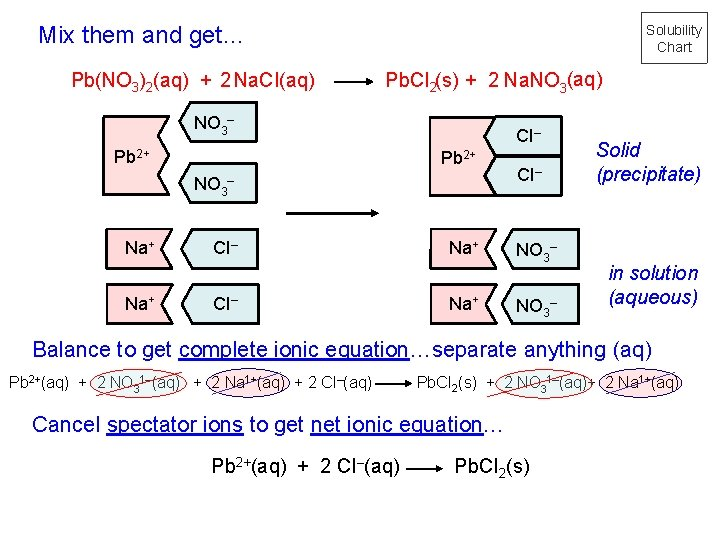Mix them and get… Pb(NO 3)2(aq) + 2 Na. CI(aq) Solubility Chart Pb. CI 2(s) + 2 Na. NO 3(aq) NO 3– Pb 2+ NO 3 Na+ CI– – CI– Na+ CI– NO 3– Solid (precipitate) in solution (aqueous) Balance to get complete ionic equation…separate anything (aq) Pb 2+(aq) + 2 NO 31–(aq) + 2 Na 1+(aq) + 2 CI–(aq) Pb. CI 2(s) + 2 NO 31–(aq)+ 2 Na 1+(aq) Cancel spectator ions to get net ionic equation… Pb 2+(aq) + 2 CI–(aq) Pb. CI 2(s)Pre-lab: 1. What ions are present in the following solutions? Na+(aq) Cl-(aq) Ag+(aq) NO 3 -(aq) Na. Cl(aq) __________ Ag. NO 3(aq) __________ 2. When these solutions are mixed together, a precipitate is seen. What are the new combinations of ions that could have formed the precipitate? Ag (aq) Na (aq) NO 3 (aq) and __________ Cl (aq) __________ + + - - 3. Using the solubility table, which new combination will form a precipitate? Ag+(aq) Cl-(aq) __________ Ag. Cl(s) 4. Which new combination will remain in solution? Na (aq) NO 3 (aq) __________ + - 5. Write the complete ionic equation for this reaction. Be sure to indicate the correct phase (reaction condition) for each reactant and each product. Na+(aq) + Cl-(aq) + Ag+(aq) + NO 3 -(aq) Ag. Cl(s) + Na+(aq) + NO 3 -(aq) 6. Write the net ionic equation for this reaction by canceling out spectators. Again, include the phases (reaction conditions). Ag+(aq) + Cl-(aq) Ag. Cl(s) 7. Explain why you would expect no reaction between solutions of KOH(aq) and Na. OH(aq). When the cations switch places they end with a hydroxide (no new combination is formed)Mix together Zn(NO 3)2(aq) and Ba(OH)2(aq): Solubility Chart Mix them and get… Zn(NO 3)2(aq) + Ba(OH)2(aq) Zn(OH)2(s) + Ba(NO 3)2(aq) Zn(NO 3)2(aq) Ba(OH)2(aq) Zn 2+(aq) + 2 NO 3–(aq) Ba 2+(aq) + 2 OH–(aq) OH– NO 3– Ba 2+ Zn 2+ NO 3– OH– Balance to get complete ionic equation… Zn 2+(aq) + 2 NO 3–(aq) + Ba 2+(aq) + 2 OH–(aq) Zn(OH)2(s) + 2 NO 3–(aq) + Ba 2+(aq) Cancel spectator ions to get net ionic equation… Zn 2+(aq) + 2 OH–(aq) Zn(OH)2(s)Separation of Cations You have a solution containing Fe 2+, Cu 2+, Ba 2+, Ag+ and K+ ions. By what means could you separate these ions from each other? • In Chem I, we discussed various ways to separate things… • • Distillation Filtration Centrifugation Reactivity Will any of these work to separate aqueous ions?Separation of Cations Fe 2+, Cu 2+, Ba 2+, Ag+, K+ (aq) + Cl- (aq) Ag. Cl(s) Fe 2+, Cu 2+, Ba 2+, K+ (aq) + SO 42 - (aq) Ba. SO 4(s) Fe 2+, Cu 2+, K+ (aq) + Cr. O 42 - (aq) Cu. Cr. O 4(s) Fe 2+, K+ (aq) + S 2 -, PO 43 - OH- or CO 32 - (aq) Fe. S, Fe 3(PO 4)2 , Fe(OH)2 , or Fe. CO 3(s) K+(aq)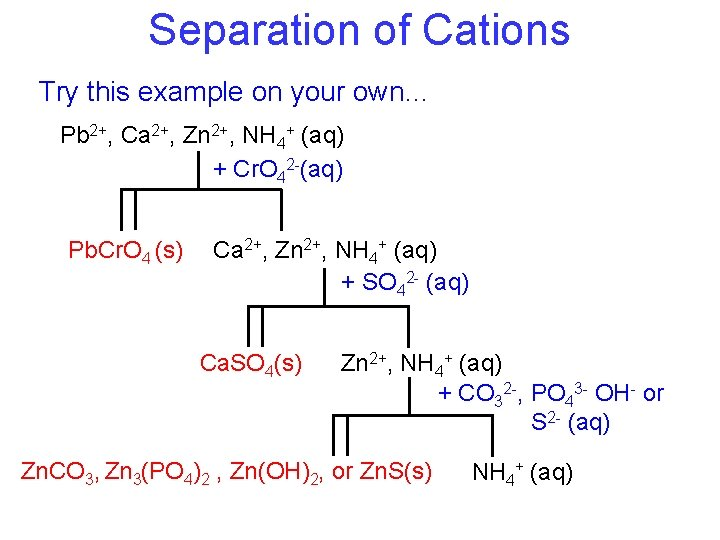Separation of Cations Try this example on your own… Pb 2+, Ca 2+, Zn 2+, NH 4+ (aq) + Cr. O 42 -(aq) Pb. Cr. O 4 (s) Ca 2+, Zn 2+, NH 4+ (aq) + SO 42 - (aq) Ca. SO 4(s) Zn 2+, NH 4+ (aq) + CO 32 -, PO 43 - OH- or S 2 - (aq) Zn. CO 3, Zn 3(PO 4)2 , Zn(OH)2, or Zn. S(s) NH 4+ (aq)Summary of Classes of Reactions Chemical reactions Double Replacement reactions Oxidation-Reduction Reactions Combustion Reactions Synthesis and Decomposition reactions Acid-Base Reactions Single Replacement reactions